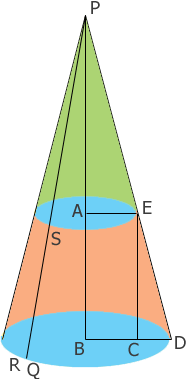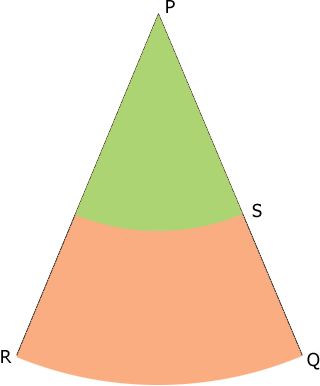SEARCH HOMEMath Central Quandaries & QueriesQuestion from John, a parent: I am trying to build a wind sock and need to be able to lay the shape out on cloth. I need the wind sock front opening (diameter) to be 3 1/2" and the rear opening diameter to be 1". The windsock needs to be 9 1/2" long. I tried using the example of the person trying to make a crayfish trap but got confused and could not figure out my numbers. Any help would be greatly appreciated. Thanks JohnHi John,

In the crayfish trap example Steve was constructing a full cone but you want a truncated cone. A better example would be Fluer's question about a lamp shade. I drew a diagram (not to scale) using the same notation as in the lampshade problem so that you can compare them.You have $|AE| = 0.5$ inches, $|BD| = 1.75$ inches and $|AB| = 9.5$ inches. Triangles $BDP$ and $CDE$ are similar and hence

$\frac{|PB|}{1.75} = \frac{9.5}{1.75 - 0.5}$

and hence

$|PB| = \frac{9.5}{1.25} \times 1.75 = 13.3 \mbox{ inches.}$

Pythagoras theorem applied to triangle $ECD$ yields

$|ED| = \sqrt{9.5^2 + 1.25^2} = 9.58 \mbox{ inches.}$

Similarly triangle $PBD$ is a right triangle so

$|PD| = \sqrt{13.3^2 + 1.75^2} = 13.42 \mbox{ inches.}$

Now slice the diagram from P to Q and roll it out to form a sector of a circle.The circumference of the circle with centre $P$ and radius $|PR| = |PD| = 13.42$ inches is $2 \pi \times 13.42$ inches and the arc from $R$ to $Q$ is the circumference of the circle that forms the base of the wind sock. Thus the length of the arc from $R$ to $Q$ is $2 \pi \times 1.75$ inches. Hence the measure of the angle $QPR$ is

$\frac{2 \pi \times 1.75}{2 \pi \times 13.42} \times 360^o = 47^o$

At this point I suggest that you check my arithmetic and then make a paper copy. You may want to make the central angle larger than $47^o$ to give some overlap in order to stitch the wind sock together.

PennyMath Central is supported by the University of Regina and The Pacific Institute for the Mathematical Sciences.